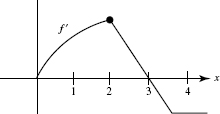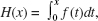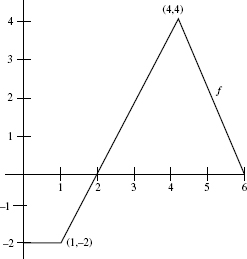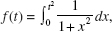# AP Calculus BC Practice Test 24

### Test Information9 questions27 minutes

Calculator Allowed

1. The path of a satellite is given by the parametric equations

x = 4 cos t + cos 12t,
y = 4 sin t + sin 12t.

The upward velocity at t = 1 equals

2. As a cup of hot chocolate cools, its temperature after t minutes is given by H(t) = 70 + ke -0.4t. If its initial temperature was 120°F, what was its average temperature (in °F) duringthe first 10 minutes?

3. An object moving along a line has velocity v (t) = t cos t - ln (t + 2), where 0 t 10. The object achieves its maximum speed when t =

4.The graph of f , which consists of a quarter-circle and two line segments, is shown above. At x = 2 which of the following statements is true?

5. Letwhere f is the function whose graph appears below.The tangent line approximating H(x) near x = 3 is H(x)6. The table shows the speed of an object, in feet per second, at various times duringa 12-second interval.

 time (sec) 0 3 6 7 8 10 12 speed (ft/sec) 15 14 11 8 7 3 0

Estimate the distance the object travels, using the midpoint method with 3 subintervals.

7. In a marathon, when the winner crosses the finish line many runners are still onthe course, some quite far behind. If the density of runners x miles from the finish line is given by R(x) = 20[1 - cos(1 + 0.03x2)] runners per mile, how many are within 8 miles of the finish line?

8. Find the volume of the solid generated when the region bounded by the y-axis, y = ex, and y = 2 is rotated around the y-axis.

9. Ifthen f (t) equals